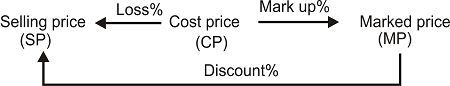# Profit and Loss Formulas

Profit loss and Discount is an application of percentages.

Cost Price: The rate at which a merchant buys goods. This is his investment

Selling Price: The rate at which a merchant sells his goods.
Marked Price: The rate at which a merchant rises his price above the cost price (may be anticipating some hagglers)

Profit Case:Loss Case:Key Formulas:

Profit or Gain = Selling Price - Cost Price = SP - CP
Loss = Cost price - Selling price = CP - SP
Profit = ${\rm{CP \times \text(Profit\% )}}$
Loss = $CP \times (Loss\% )$
Profit % = $\displaystyle\frac{{SP - CP}}{{CP\strut}} \times 100 = \frac{{Profit}}{{CP\strut}} \times 100$
Loss % = $\displaystyle\frac{{CP - SP}}{{CP\strut}} \times 100 = \frac{{Loss}}{{CP\strut}} \times 100$
Important: Profit or Loss always calculated on Cost price Only.

Discount = Marked price - Selling Price = MP - SP

Discount % = $\displaystyle\frac{{MP - SP}}{{MP}} \times 100 = \frac{{Discount}}{{MP}} \times 100$

Calculating Selling price from Cost price:

In the profit case selling price is greater than cost price, and this case we gain some profit.  That is we are increasing the cost price by some percentage to get the selling price.  This can be done in several ways

In profit case

$CP + CP \times (\Pr ofit\% )$ = SP
$CP \times (100 + \Pr ofit)\%$ = SP
(V.imp: To get the selling price we have to multiply the cost price by (100+x)% if profit percent = x%)

In loss case

$CP - CP \times Loss\%$=SP
$CP \times (100 - Loss)\%$ = SP
(V.imp: To get the selling price we have to multiply the cost price by (100-x)% if loss percent = x%)

Calculating Selling Price from Marked Price:

$MP - MP \times discount\%$ = SP
$MP \times \left( {100 - discount} \right)\%$ = SP

Calculating Cost price from Selling Price:

This is the reverse operation of the above
In profit case: $\displaystyle\frac{{SP}}{{(100 + Profit)\% }} = CP$
In loss Case: $\displaystyle\frac{{SP}}{{(100 - Loss)\% }} = CP$
(V.imp: To get the cost price we have to divide the selling price by (100+x)% in profit case and by (100-x)% in loss case)